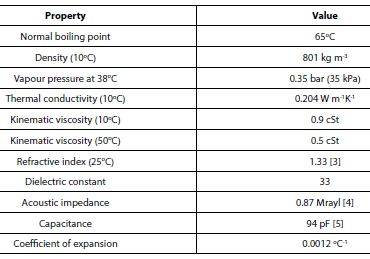Chemistry

# Methanol Alcohol Containing Fuels

As methanol is a pure chemical compound its physical properties are precise. For example, there is no need to talk of the Reid Vapour Pressure (RVP) of methanol as there is no dependence of the vapour pressure on the space into which the vapour expands as there is with gasoline. Selected physical properties of methanol liquid are given in the table below having been taken from unless otherwise indicated.ln [ⱱ(T2)/ⱱ(T1)] = ΔE/R {(1/T2) — (1/T1)}

ΔE = 11 kJ mol-1

The heat of vaporisation in the temperature range of interest is actually 34 kJ mol-1, and ΔE is 32% of this so there is good conformity. There is scope for enhancing the precision of the calculation by avoiding the implicit assumption that the density variation over the temperature range is negligible (that is, by using the ratio of dynamic viscosities instead of the ratio of kinematic viscosities).